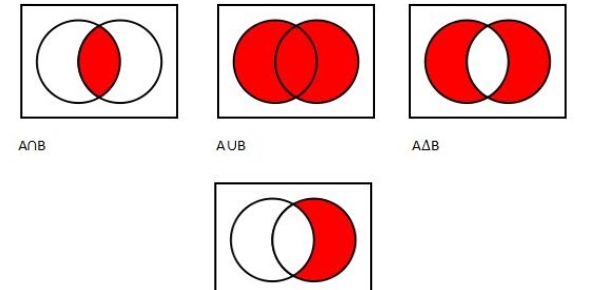# Set Theory And Logic Quiz

Approved & Edited by ProProfs Editorial Team
At ProProfs Quizzes, our dedicated in-house team of experts takes pride in their work. With a sharp eye for detail, they meticulously review each quiz. This ensures that every quiz, taken by over 100 million users, meets our standards of accuracy, clarity, and engagement.
| Written by Talanti
T
Talanti
Community Contributor
Quizzes Created: 1 | Total Attempts: 9,410
Questions: 10 | Attempts: 9,477SettingsDo you understand the concept of logic and set theory? Take this set theory quiz, and see how well you understand this concept. The process of derivation of properties is called logic. The general properties of sets and elements are called the set theory. Here, we have logic and set theory questions and answers to test your knowledge. All the best! You can share the quiz with others interested in solving math problems.

• 1.

### A set is:

• A.

Collection of objects

• B.

Well-defined collection of objects

• C.

Elements of the set

• D.

Description

• E.

Collection

B. Well-defined collection of objects
Explanation
A set is a well-defined collection of objects. This means that a set must have clear and unambiguous criteria for determining whether an object belongs to the set or not. It cannot be vague or subjective. The objects in a set are referred to as elements of the set. Therefore, a set is a well-defined collection of objects where each object is an element of the set.

Rate this question:

• 2.

### The symbol  ∈ means:

• A.

Is not an element of

• B.

Is description

• C.

Is an element of

• D.

Does not belong

C. Is an element of
Explanation
The symbol "∈" is used in set theory to indicate that an element belongs to a set. It represents the concept of membership, indicating that the element is part of the set.

Rate this question:

• 3.

### For larger sets:

• A.

The description method is used, and it is more convenient

• B.

The description method is not used

• C.

The description method is never used

• D.

All of the above

A. The description method is used, and it is more convenient
Explanation
The correct answer is "the description method is used, and it is more convenient." This means that for larger sets, the description method is utilized because it is considered to be more convenient. This suggests that the description method is preferred and offers advantages over other methods when dealing with larger sets.

Rate this question:

• 4.

### Z = {. . . ,−2,−1, 0, 1, 2, . . .} is the set of integers.

• A.

True

• B.

False

A. True
Explanation
The statement is true because the set Z includes all integers, which are whole numbers both positive and negative, as well as zero. It is represented by the ellipsis notation, which indicates that the set continues indefinitely in both the negative and positive directions. Therefore, the given statement accurately describes the set of integers.

Rate this question:

• 5.

### N = {0, 1, 2, 3, . . .} is the set of integers.

• A.

True

• B.

False

A. True
Explanation
The statement is true because N represents the set of integers, which includes all whole numbers from 0 to positive infinity. Therefore, the set N does indeed include the numbers 0, 1, 2, 3, and so on.

Rate this question:

• 6.

### All elements of all the sets under discussion belong to some universal set or universe.

• A.

True

• B.

False

A. True
Explanation
This statement is true because in set theory, all sets are considered to be subsets of a universal set. The universal set contains all the elements that are being discussed in the context of the sets. Therefore, all elements of all the sets under discussion do indeed belong to some universal set.

Rate this question:

• 7.

### The empty set is the set containing no elements.

• A.

True

• B.

False

A. True
Explanation
The empty set, also known as the null set, is a set that does not contain any elements. It is denoted by the symbol Ø or {} and is considered a subset of every set. Therefore, the statement "The empty set is the set containing no elements" is true.

Rate this question:

• 8.

### Let A and B be sets. A equals B, written A=B, iff every element of A is also an element of B, and conversely, every element of B is also an element of A.

• A.

True

• B.

False

A. True
Explanation
The statement is true because it accurately defines the concept of set equality. According to the definition, two sets A and B are equal if and only if every element in A is also in B, and vice versa. This means that if A equals B, then any element that belongs to A must also belong to B, and any element that belongs to B must also belong to A. Therefore, the correct answer is true.

Rate this question:

• 9.

### Theorem. There is only one empty set, i.e., only two empty sets are equal.

• A.

True

• B.

False

A. True
Explanation
This statement is true because the empty set is a unique set with no elements. It is defined as a set that contains no elements, and by definition, there can only be one set that meets this criteria. Therefore, there is only one empty set and any other set that is empty is equal to it.

Rate this question:

• 10.

### Let A and B be sets. Then A=B iff A belongs to B.

• A.

True

• B.

FalseBack to top